# How to plot fitted lines with ggplot2

Want to share your content on R-bloggers? click here if you have a blog, or here if you don't.

Most analyses aren’t really done until we’ve found a way to visualize the results graphically, and I’ve recently been getting some questions from students on how to plot fitted lines from models. There are some R packages that are made specifically for this purpose; see packages effects and visreg, for example.

If using the ggplot2 package for plotting, fitted lines from simple models can be graphed using `geom_smooth()`. However, once models get more complicated that convenient function is no longer useful. I’ll go over the approach that I use for plotting fitted lines in ggplot2 that can be used across many model types and situations.

First I’ll load the packages I’m using today.

``````library(nlme) # v. 3.1-137
library(ggplot2) # v. 3.1.0``````

I’m going to set the ggplot2 theme to `theme_bw()`.

``theme_set(theme_bw())``

I created a dataset to use for fitting models and used `dput()` to copy and paste it here.

``````dat = structure(list(block = structure(c(1L, 1L, 1L, 1L, 2L, 2L, 2L,
2L, 3L, 3L, 3L, 3L, 4L, 4L, 4L, 4L, 5L, 5L, 5L, 5L, 6L, 6L, 6L,
6L, 7L, 7L, 7L, 7L, 8L, 8L, 8L, 8L, 9L, 9L, 9L, 9L, 10L, 10L,
10L, 10L), .Label = c("A", "B", "C", "D", "E", "F", "G", "H",
"I", "J"), class = "factor"), grp = structure(c(1L, 2L, 3L,
4L, 1L, 2L, 3L, 4L, 1L, 2L, 3L, 4L, 1L, 2L, 3L, 4L, 1L, 2L, 3L,
4L, 1L, 2L, 3L, 4L, 1L, 2L, 3L, 4L, 1L, 2L, 3L, 4L, 1L, 2L, 3L,
4L, 1L, 2L, 3L, 4L), .Label = c("a", "b", "c", "d"), class = "factor"),
x1 = c(11.1, 7.9, 6.6, 7.1, 10.6, 8, 9.8, 8.2, 9.5, 5.4,
15, 15.3, 10.4, 5.5, 9.1, 15.3, 12.9, 9.9, 5, 7.4, 10.4,
8.2, 14.1, 4.7, 11.9, 12.5, 8.7, 7, 5.5, 5.7, 13.7, 11.8,
7, 14.8, 4.9, 14.3, 7.8, 15.4, 15.2, 12.2), x2 = c(109.9,
149.2, 187.4, 124.1, 190.7, 145, 110.1, 114.1, 119.9, 163.8,
192.7, 158.3, 180.5, 127.7, 133.1, 137.5, 167.8, 181.8, 156.4,
109.7, 143.9, 194.2, 139.1, 112.4, 194, 125.7, 127, 149.1,
117.8, 170.4, 167.3, 101.1, 128, 157.8, 139.7, 193.6, 121.1,
161.1, 112, 137.3), resp = c(86.5, 63.1, 10.5, 44.4, 61.9,
67.7, 64.1, 59.4, 66.1, 33.2, 91.6, 116.4, 59.4, 38.6, 44.6,
122.9, 87.1, 75.1, -0.8, 49.1, 70.2, 57.8, 96.4, 22.5, 74.7,
116.7, 46, 39.8, 28.3, 34.1, 87, 97.1, 37.3, 126.8, 2.2,
96.1, 45.3, 131.9, 107.6, 92.7)), class = "data.frame", row.names = c(NA,
-40L))``````

This dataset has one response variable, `resp`, along with two continuous (`x1`, `x2`) and one categorical (`grp`) explanatory variables. These data are from a blocked design, and the `block` variable is available to be used as a random effect.

``head(dat)``
``````#   block grp   x1    x2 resp
# 1     A   a 11.1 109.9 86.5
# 2     A   b  7.9 149.2 63.1
# 3     A   c  6.6 187.4 10.5
# 4     A   d  7.1 124.1 44.4
# 5     B   a 10.6 190.7 61.9
# 6     B   b  8.0 145.0 67.7``````

# Plotting separate slopes with geom_smooth()

The `geom_smooth()` function in ggplot2 can plot fitted lines from models with a simple structure. Supported model types include models fit with `lm()`, `glm()`, `nls()`, and `mgcv::gam()`.

Fitted lines can vary by groups if a factor variable is mapped to an aesthetic like `color` or `group`. I’m going to plot fitted regression lines of `resp` vs `x1` for each `grp` category.

By default you will get confidence intervals plotted in `geom_smooth()`. This can be great if you are plotting the results after you’ve checked all assumptions but is not-so-great if you are exploring the data. Confidence intervals can be suppressed using `se = FALSE`, which I use below.

This is a linear model fit, so I use `method = "lm"`.

``````ggplot(dat, aes(x = x1, y = resp, color = grp) ) +
geom_point() +
geom_smooth(method = "lm", se = FALSE)``````Here is the same plot with a 95% confidence envelope (the default interval size) as a ribbon around the fitted lines. I used `fill` to make the ribbons the same color as the lines. I increased the transparency of the ribbons by decreasing `alpha`, as well, since adding confidence ribbons for many fitted lines in one plot can end up looking pretty messy.

``````ggplot(dat, aes(x = x1, y = resp, color = grp) ) +
geom_point() +
geom_smooth(method = "lm", alpha = .15, aes(fill = grp))``````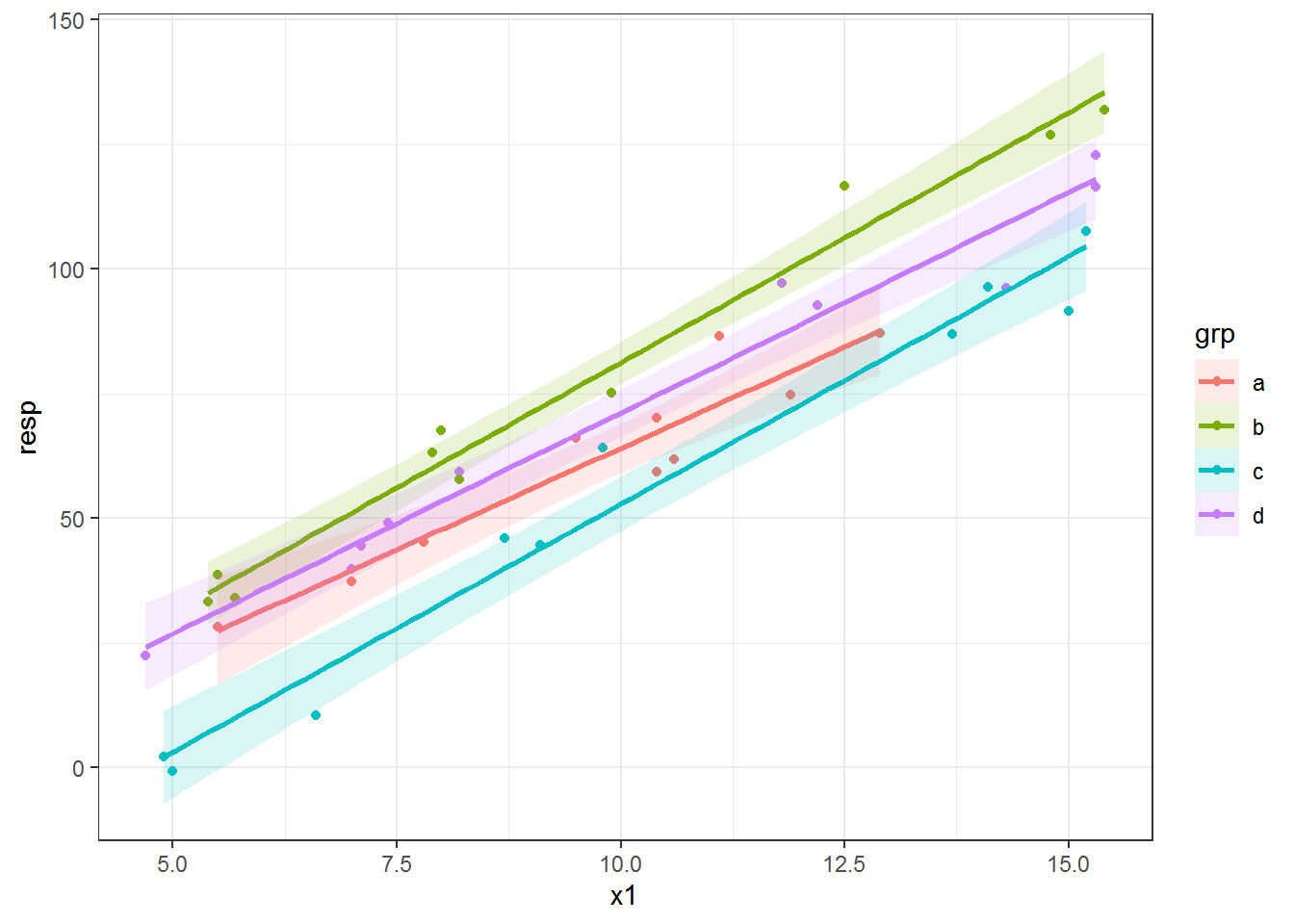# Extracting predicted values with predict()

In the plots above you can see that the slopes vary by `grp` category. If you want parallel lines instead of separate slopes per group, `geom_smooth()` isn’t going to work for you.

To free ourselves of the constraints of `geom_smooth()`, we can take a different plotting approach. We can instead fit a model and extract the predicted values. These predicted values can then be used for drawing the fitted line(s). For many model types the predictions can be extracted from the fitted model via the `predict()` function.

You can check if the model you are using has a predict function via `methods()`. For example, `methods("predict")` lists all the different model objects that have specific `predict()` functions. Since I’ve already loaded package nlme you can see `predict.lme` and `predict.gls` along with many others. (Also see, e.g., `methods(class = "lm")` for functions available for a specific model type.)

``methods("predict")``
``````#   predict.ar*                predict.Arima*
#   predict.arima0*            predict.glm
#   predict.gls*               predict.gnls*
#   predict.HoltWinters*       predict.lm
#   predict.lme*               predict.lmList*
#  predict.loess*             predict.mlm*
#  predict.nlme*              predict.nls*
#  predict.poly*              predict.ppr*
#  predict.prcomp*            predict.princomp*
#  predict.smooth.spline*     predict.smooth.spline.fit*
#  predict.StructTS*
# see '?methods' for accessing help and source code``````

You can go to the help page for the `predict()` function for a specific model type. For example, `?predict.lme` will take you to the documentation for the `predict()` function for `lme` objects fit with `nlme::lme()`.

# Plotting predicted values with geom_line()

The first step of this “prediction” approach to plotting fitted lines is to fit a model. I’ll use a linear model with a different intercept for each `grp` category and a single `x1` slope to end up with parallel lines per group.

``fitlm = lm(resp ~ grp + x1, data = dat)``

I can add the predicted values to the dataset.

``dat\$predlm = predict(fitlm)``

And then use these in `geom_line()` to add fitted lines based on the new `predlm` variable.

``````ggplot(dat, aes(x = x1, y = resp, color = grp) ) +
geom_point() +
geom_line(aes(y = predlm), size = 1)``````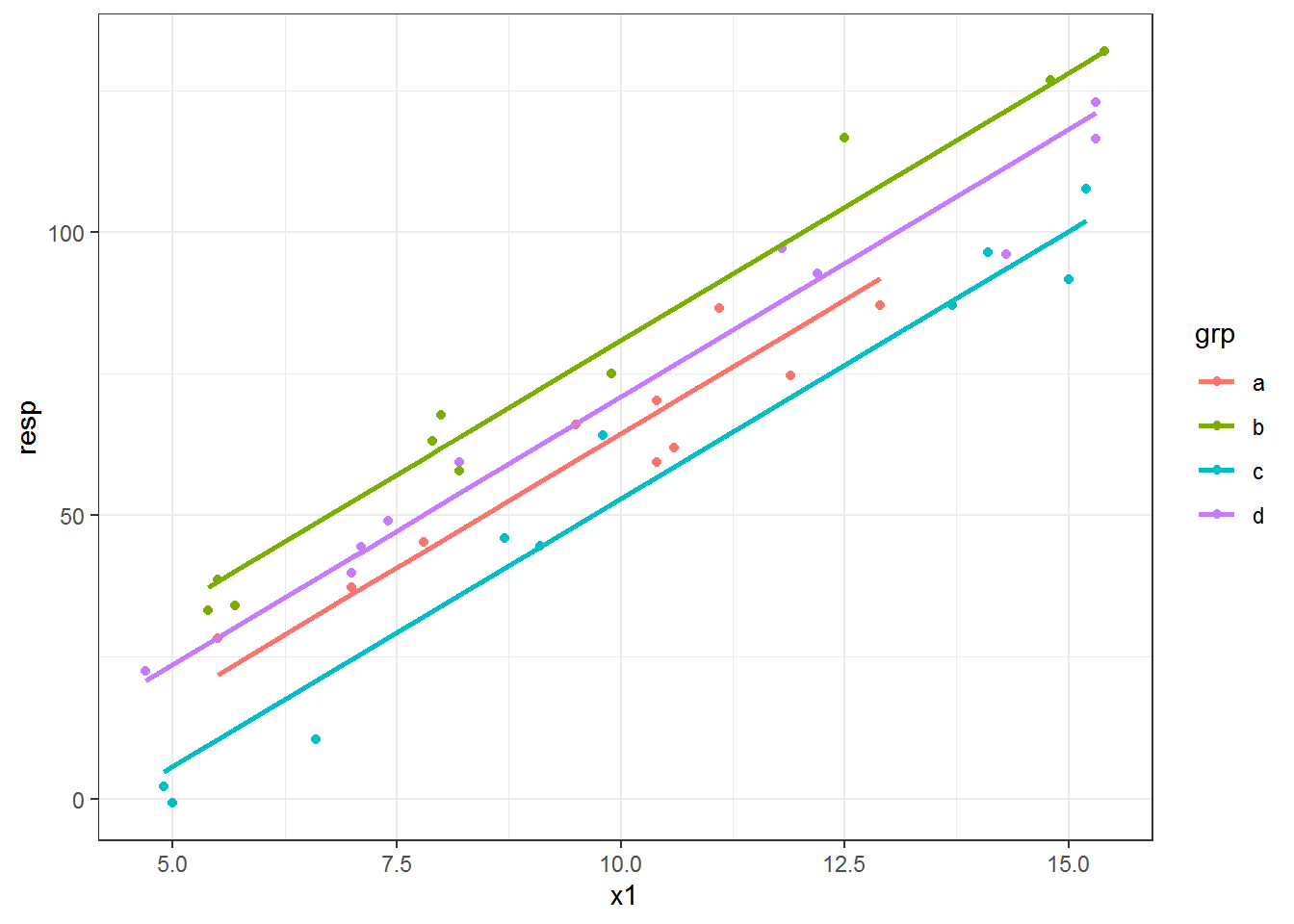# Add confidence intervals for lm objects

What about confidence intervals? The `predict()` function for `lm` objects has an `interval` argument that returns confidence or prediction intervals, which are appropriate to use if model assumptions have been reasonably met. I’m skipping the assumption-checking step here. 😉

Adding `interval = "confidence"` returns a three column matrix, where `fit` contains the fitted values and `lwr` and `upr` contain the lower and upper confidence interval limits of the predicted values, respectively. I used the default and so get a 95% confidence interval for each predicted value.

``````predslm = predict(fitlm, interval = "confidence")
``````#        fit      lwr      upr
# 1 74.81834 70.34137 79.29530
# 2 60.98358 56.50132 65.46584
# 3 20.79813 15.82711 25.76916
# 4 43.50991 38.65007 48.36975
# 5 70.09232 65.66922 74.51542
# 6 61.92879 57.45949 66.39808``````

These columns can be bound to `dat` for plotting.

``````datlm = cbind(dat, predslm)
``````#   block grp   x1    x2 resp   predlm      fit      lwr      upr
# 1     A   a 11.1 109.9 86.5 74.81834 74.81834 70.34137 79.29530
# 2     A   b  7.9 149.2 63.1 60.98358 60.98358 56.50132 65.46584
# 3     A   c  6.6 187.4 10.5 20.79813 20.79813 15.82711 25.76916
# 4     A   d  7.1 124.1 44.4 43.50991 43.50991 38.65007 48.36975
# 5     B   a 10.6 190.7 61.9 70.09232 70.09232 65.66922 74.51542
# 6     B   b  8.0 145.0 67.7 61.92879 61.92879 57.45949 66.39808``````

Now we can plot the lines using `geom_line()` and add a confidence envelope via `geom_ribbon()`. Note I have to use an `alpha` value less than 1 to make the ribbon transparent. I put the ribbon layer before the line in the plot so the line is drawn on top of the ribbon.

The `color` aesthetic affects the ribbon outline, which I didn’t really like. I used `color = NULL` to remove the outlines all together and then mapped the `grp` variable to the `fill` aesthetic. If I wanted gray ribbons instead I could have used the `group` aesthetic in place of `fill`.

``````ggplot(datlm, aes(x = x1, y = resp, color = grp) ) +
geom_point() +
geom_ribbon( aes(ymin = lwr, ymax = upr, fill = grp, color = NULL), alpha = .15) +
geom_line( aes(y = fit), size = 1)``````# Using a new dataset with predict()

The fitted lines in all the plots so far are different lengths. This is because we have slightly different ranges of `x1` for each `grp` category in the dataset. By default when using `predict()` we get the fitted values; i.e., the predicted values from the dataset used in model fitting.

I think having different line lengths is fine here, but there are times when we want to draw each line across the entire range of the variable in the dataset. Also, sometimes our data are so sparse that our fitted line ends up not being very smooth; this can be especially problematic for non-linear fits. In both of these situations we’d want to make a new dataset for making the predictions.

Let’s make group lines using the entire range of `x1` instead of the within-group range. We can make a variable with the full range of `x1` via `seq()`, making a sequence from the minimum to maximum dataset value. I use 0.1 as the increment in `seq()`; the increment value you’ll want to use depends on the range of your variable.

``head( seq(min(dat\$x1), max(dat\$x1), by = .1) )``
``#  4.7 4.8 4.9 5.0 5.1 5.2``

Then to get this full range `x1` associated with each `grp` category we can use `expand.grid()`.

``````newdat = expand.grid(x1 = seq(min(dat\$x1), max(dat\$x1), by = .1),
grp = unique(dat\$grp) )``````

The key to making a dataset for prediction is that it must have every variable used in the model in it. You will get an error if you forget a variable or make a typo in one of the variable names. Note that the prediction dataset does not need to contain the response variable.

We use this prediction dataset with the `newdata` argument in `predict()`. I’ll add the predicted values as a new variable to the prediction dataset.

``newdat\$predlm = predict(fitlm, newdata = newdat)``

When we make the plot of the fitted lines now we can see that the line for each group covers the same range. There are now two datasets used in the plotting code: the original for the points and `newdat` within `geom_line()`.

``````ggplot(dat, aes(x = x1, y = resp, color = grp) ) +
geom_point() +
geom_line(data = newdat, aes(y = predlm), size = 1)``````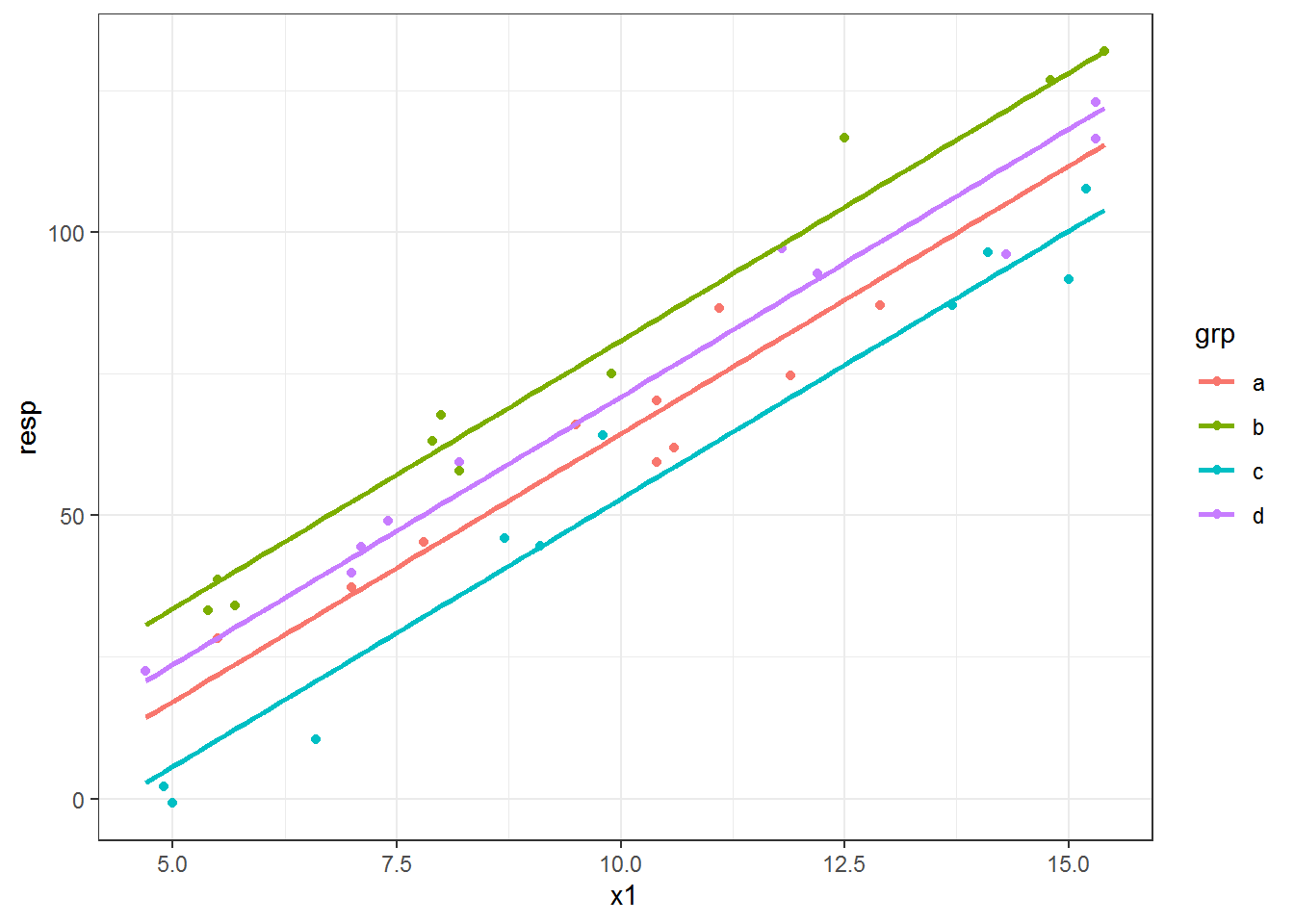# Plotting fitted lines from an lme object

The approach I demonstrated above, where the predicted values are extracted and used for plotting the fitted lines, works across many model types and is the general approach I use for most fitted line plotting I do in ggplot2.

I’ll show one more example, this time using the “real” model. 😀 This is the model that I used to create `resp`. The model is a linear mixed model with all three explanatory variables as additive fixed effects (no interactions) along with the random effect of `block`.

``````fitlme = lme(resp ~ grp + x1 + x2,
random = ~1|block,
data = dat)``````

We can make predictions via the `predict()` function for `lme` objects. However, since I have two continuous explanatory variables I’ll have to do this for one variable while holding the other fixed. This is called an added variable plot, which I’ve written about before.

I’ll focus on making a plot for `x1` while holding `x2` at its median. I’m going to make a new dataset for prediction since `x2` will be a constant.

I could make a sequence for `x1` like I did above, but instead I simply pull `grp` and `x1` from the original dataset. Since I don’t want to use the random effect in my predictions I do not include `block` in this prediction dataset.

``````newdat.lme = data.frame(grp = dat\$grp,
x1 = dat\$x1,
x2 = median(dat\$x2) )
``````#   grp   x1    x2
# 1   a 11.1 141.8
# 2   b  7.9 141.8
# 3   c  6.6 141.8
# 4   d  7.1 141.8
# 5   a 10.6 141.8
# 6   b  8.0 141.8``````

I use `level = 0` in `predict()` to get the marginal or population predictions (this is equivalent to `re.form = NA` for lme4 models). See `?predict.lme` for more info.

If I wanted to make conditional predictions, `block` would need to be part of `newdat.lme`. Conditional predictions would not get you nice straight lines for the overall fixed effects. 😜

``newdat.lme\$predlme = predict(fitlme, newdata = newdat.lme, level = 0)``

Since this is an added variable plot (from a model with multiple continuous variables), it doesn’t make a lot of sense to plot the line directly on top of the raw data points. I switch to using a rug plot for the `x` axis so we can see where we have data.

``````ggplot(dat, aes(x = x1, y = resp, color = grp) ) +
geom_rug(sides = "b", size = 1) +
geom_line(data = newdat.lme, aes(y = predlme), size = 1)``````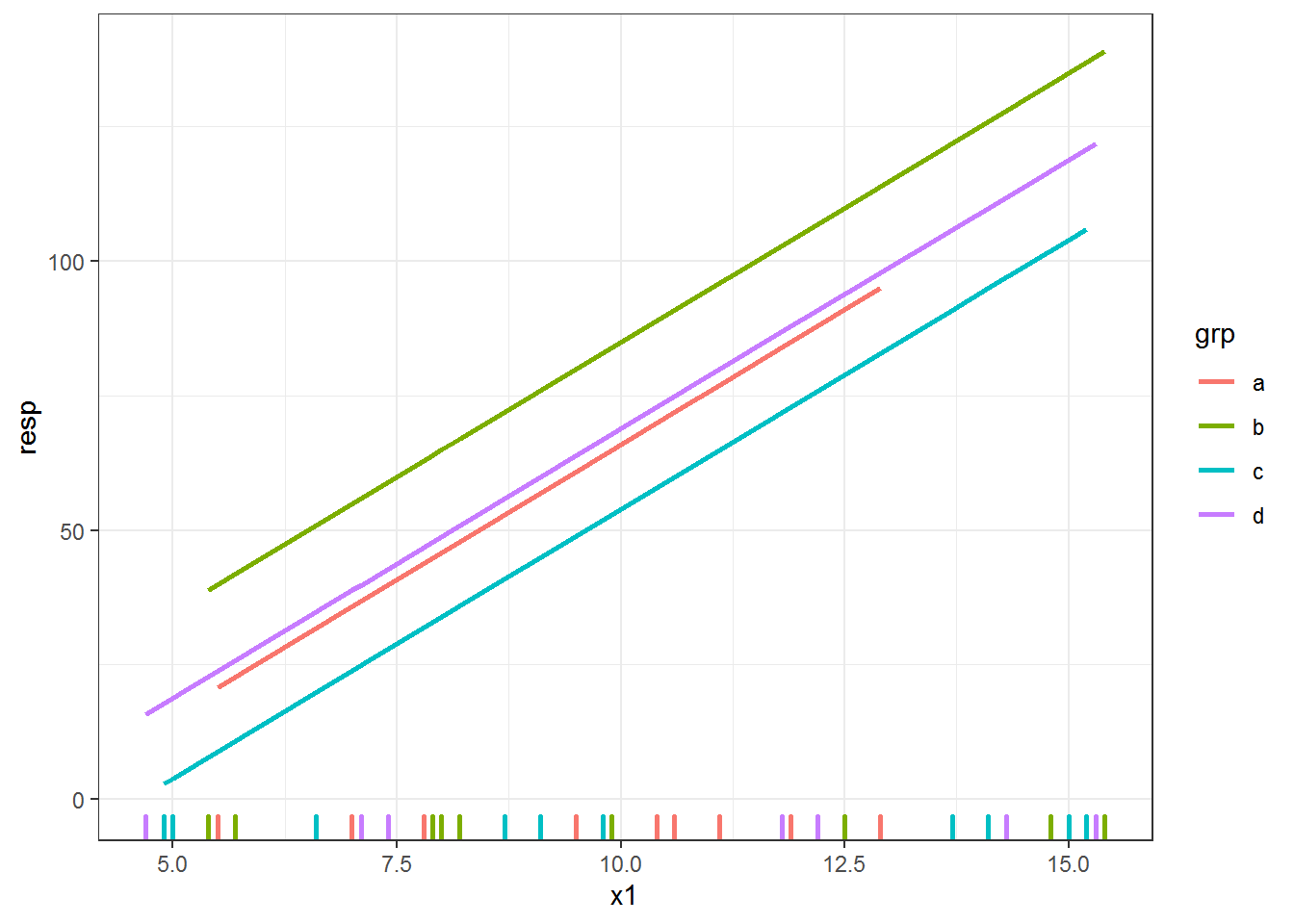# Confidence intervals for lme objects

What if we wanted to add a confidence envelope? You’ll see `predict.lme` does not have an option to get confidence intervals or calculate standard errors that could be used to build confidence intervals. I use the recipe from the GLMM FAQ maintained by Ben Bolker, although this approach does not take the uncertainty of the random effects into account.

This approach involves getting the model matrix \(X\), the covariance matrix of the parameters \(V\), and calculating \(XVX'\).

First we get the model matrix using the prediction dataset. The code looks extra complicated because we don’t have `resp` in the prediction dataset.

``des = model.matrix(formula(fitlme)[-2], newdat.lme)``

Then we use matrix multiplication on the model matrix and variance-covariance matrix extracted from the model with `vcov()`. We pull out the values on the diagonal, which are the variances of the predicted values.

``predvar = diag( des %*% vcov(fitlme) %*% t(des) )``

To construct approximate confidence intervals we can use the standard errors (square root of `predvar`) along with an appropriate multiplier. I’m using 2 as a multiplier, but you could also figure out the appropriate \(t\) multiplier based on the degrees of freedom or use 1.96 as a \(z\) multiplier.

I add the confidence interval limits to the dataset for plotting.

``````newdat.lme\$lower = with(newdat.lme, predlme - 2*sqrt(predvar) )
newdat.lme\$upper = with(newdat.lme, predlme + 2*sqrt(predvar) )``````

Here’s the plot, with a (very small!) confidence envelope for each line.

``````ggplot(dat, aes(x = x1, y = resp, color = grp) ) +
geom_rug(sides = "b", size = 1) +
geom_ribbon(data = newdat.lme, aes(y = NULL, ymin = lower, ymax = upper,
color = NULL, fill = grp),
alpha = .15) +
geom_line(data = newdat.lme, aes(y = predlme), size = .75)``````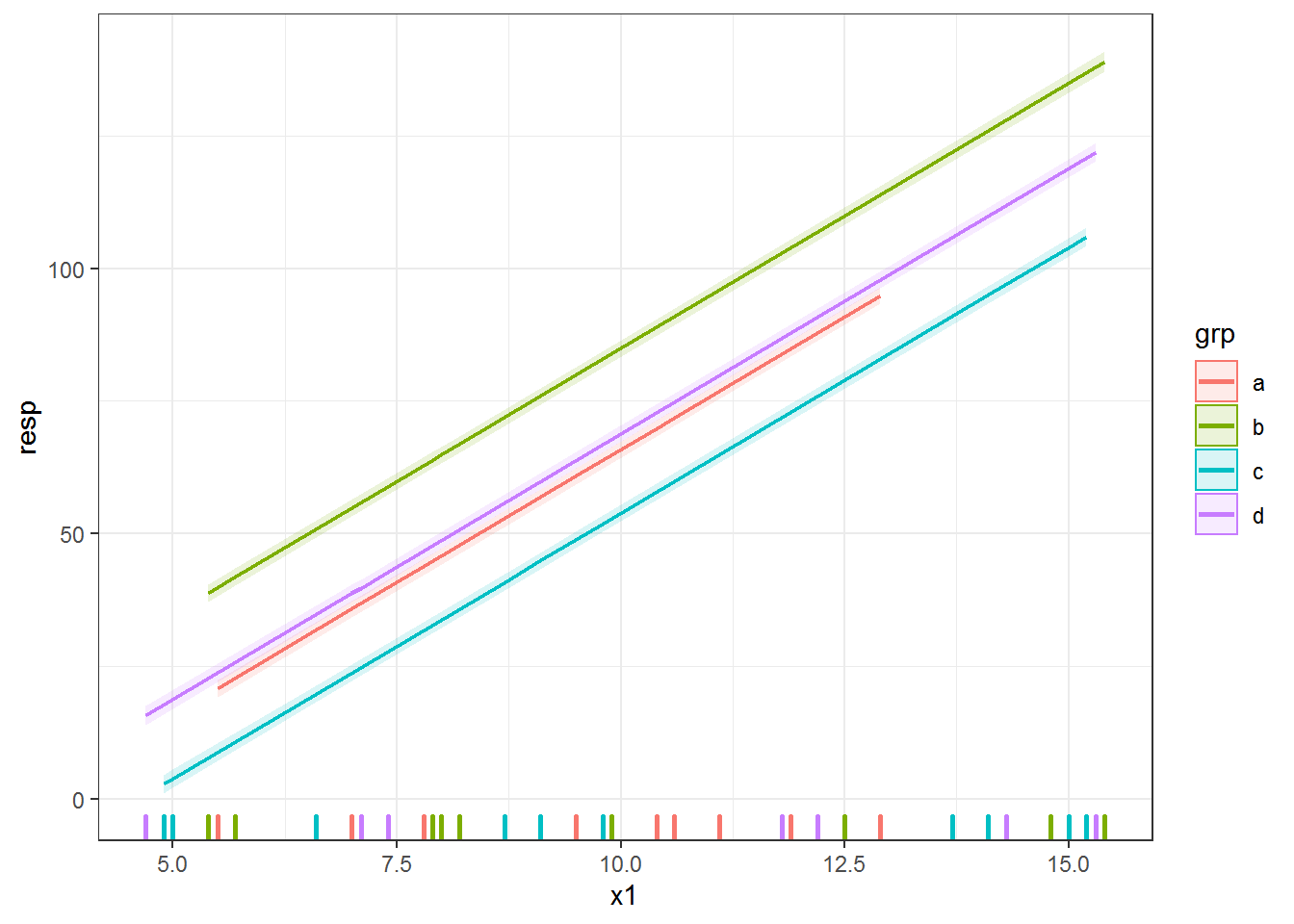# What if there is no predict() function?

In my experience, the vast majority of modeling packages these days have `predict()` functions. If the one you are using doesn’t, though, you can usually do your own predictions with matrix multiplication of the model matrix and the fixed effects. You can see an example for the glmmADMB package from the GLMM FAQ here.

Here’s the code without all the discussion. Copy and paste the code below or you can download an R script of uncommented code from here.

``````library(nlme) # v. 3.1-137
library(ggplot2) # v. 3.1.0

theme_set(theme_bw())

dat = structure(list(block = structure(c(1L, 1L, 1L, 1L, 2L, 2L, 2L,
2L, 3L, 3L, 3L, 3L, 4L, 4L, 4L, 4L, 5L, 5L, 5L, 5L, 6L, 6L, 6L,
6L, 7L, 7L, 7L, 7L, 8L, 8L, 8L, 8L, 9L, 9L, 9L, 9L, 10L, 10L,
10L, 10L), .Label = c("A", "B", "C", "D", "E", "F", "G", "H",
"I", "J"), class = "factor"), grp = structure(c(1L, 2L, 3L,
4L, 1L, 2L, 3L, 4L, 1L, 2L, 3L, 4L, 1L, 2L, 3L, 4L, 1L, 2L, 3L,
4L, 1L, 2L, 3L, 4L, 1L, 2L, 3L, 4L, 1L, 2L, 3L, 4L, 1L, 2L, 3L,
4L, 1L, 2L, 3L, 4L), .Label = c("a", "b", "c", "d"), class = "factor"),
x1 = c(11.1, 7.9, 6.6, 7.1, 10.6, 8, 9.8, 8.2, 9.5, 5.4,
15, 15.3, 10.4, 5.5, 9.1, 15.3, 12.9, 9.9, 5, 7.4, 10.4,
8.2, 14.1, 4.7, 11.9, 12.5, 8.7, 7, 5.5, 5.7, 13.7, 11.8,
7, 14.8, 4.9, 14.3, 7.8, 15.4, 15.2, 12.2), x2 = c(109.9,
149.2, 187.4, 124.1, 190.7, 145, 110.1, 114.1, 119.9, 163.8,
192.7, 158.3, 180.5, 127.7, 133.1, 137.5, 167.8, 181.8, 156.4,
109.7, 143.9, 194.2, 139.1, 112.4, 194, 125.7, 127, 149.1,
117.8, 170.4, 167.3, 101.1, 128, 157.8, 139.7, 193.6, 121.1,
161.1, 112, 137.3), resp = c(86.5, 63.1, 10.5, 44.4, 61.9,
67.7, 64.1, 59.4, 66.1, 33.2, 91.6, 116.4, 59.4, 38.6, 44.6,
122.9, 87.1, 75.1, -0.8, 49.1, 70.2, 57.8, 96.4, 22.5, 74.7,
116.7, 46, 39.8, 28.3, 34.1, 87, 97.1, 37.3, 126.8, 2.2,
96.1, 45.3, 131.9, 107.6, 92.7)), class = "data.frame", row.names = c(NA,
-40L))

ggplot(dat, aes(x = x1, y = resp, color = grp) ) +
geom_point() +
geom_smooth(method = "lm", se = FALSE)

ggplot(dat, aes(x = x1, y = resp, color = grp) ) +
geom_point() +
geom_smooth(method = "lm", alpha = .15, aes(fill = grp))

methods("predict")

fitlm = lm(resp ~ grp + x1, data = dat)
dat\$predlm = predict(fitlm)

ggplot(dat, aes(x = x1, y = resp, color = grp) ) +
geom_point() +
geom_line(aes(y = predlm), size = 1)

predslm = predict(fitlm, interval = "confidence")

datlm = cbind(dat, predslm)

ggplot(datlm, aes(x = x1, y = resp, color = grp) ) +
geom_point() +
geom_ribbon( aes(ymin = lwr, ymax = upr, fill = grp, color = NULL), alpha = .15) +
geom_line( aes(y = fit), size = 1)

head( seq(min(dat\$x1), max(dat\$x1), by = .1) )

newdat = expand.grid(x1 = seq(min(dat\$x1), max(dat\$x1), by = .1),
grp = unique(dat\$grp) )

newdat\$predlm = predict(fitlm, newdata = newdat)

ggplot(dat, aes(x = x1, y = resp, color = grp) ) +
geom_point() +
geom_line(data = newdat, aes(y = predlm), size = 1)

fitlme = lme(resp ~ grp + x1 + x2,
random = ~1|block,
data = dat)

newdat.lme = data.frame(grp = dat\$grp,
x1 = dat\$x1,
x2 = median(dat\$x2) )

newdat.lme\$predlme = predict(fitlme, newdata = newdat.lme, level = 0)

ggplot(dat, aes(x = x1, y = resp, color = grp) ) +
geom_rug(sides = "b", size = 1) +
geom_line(data = newdat.lme, aes(y = predlme), size = 1)

des = model.matrix(formula(fitlme)[-2], newdat.lme)

predvar = diag( des %*% vcov(fitlme) %*% t(des) )

newdat.lme\$lower = with(newdat.lme, predlme - 2*sqrt(predvar) )
newdat.lme\$upper = with(newdat.lme, predlme + 2*sqrt(predvar) )

ggplot(dat, aes(x = x1, y = resp, color = grp) ) +
geom_rug(sides = "b", size = 1) +
geom_ribbon(data = newdat.lme, aes(y = NULL, ymin = lower, ymax = upper,
color = NULL, fill = grp),
alpha = .15) +
geom_line(data = newdat.lme, aes(y = predlme), size = .75)``````

R-bloggers.com offers daily e-mail updates about R news and tutorials about learning R and many other topics. Click here if you're looking to post or find an R/data-science job.
Want to share your content on R-bloggers? click here if you have a blog, or here if you don't.